Question

# Oxygen is composed of three isotopes: oxygen-16, oxygen-17, oxygen-18 and has an average atomic mass of...

Oxygen is composed of three isotopes: oxygen-16, oxygen-17, oxygen-18 and has an average atomic mass of 15.9992 amu. Oxygen-17 has a mass of 16.980 amu and makes up 0.033% of oxygen. Oxygen-16 has a mass of 15.988 amu and oxygen-18 has a mass of 17.984 amu. What is the percent abundance of oxygen-18?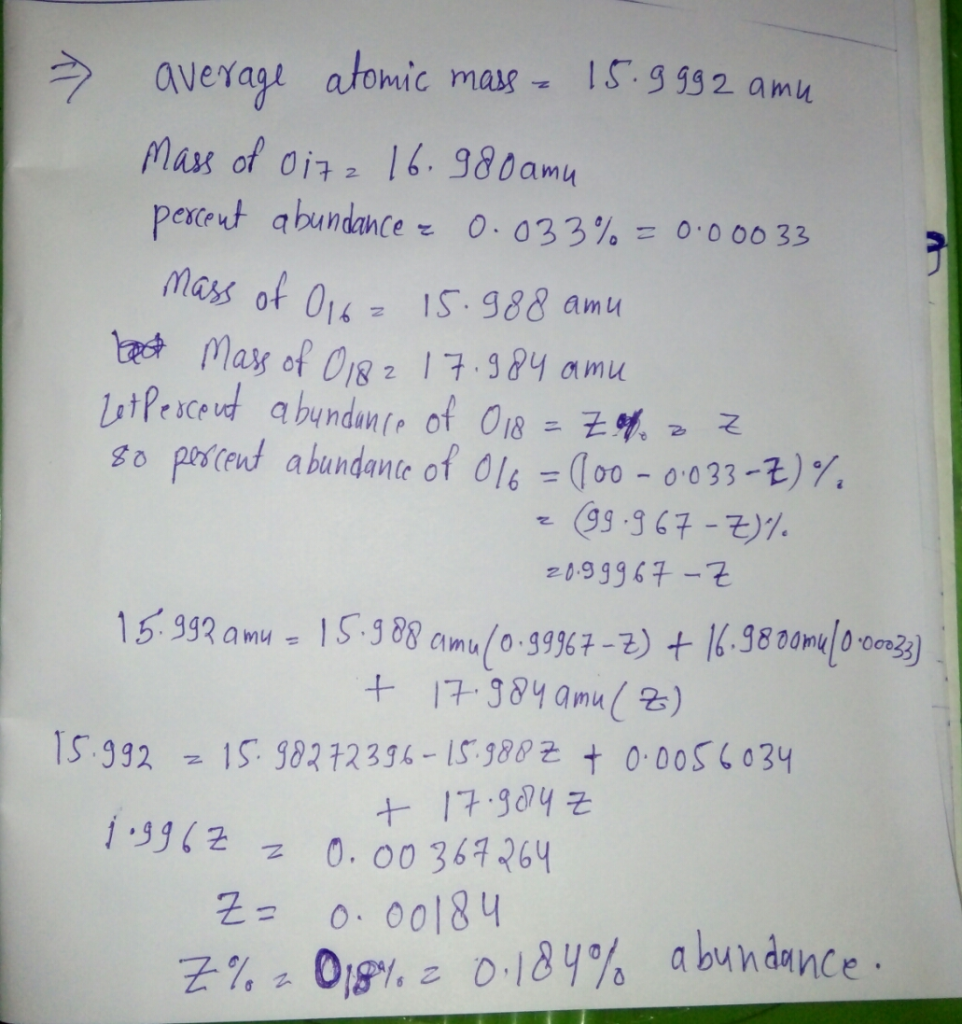...........

If you have any questions please feel free to put down in comment box..

#### Earn Coins

Coins can be redeemed for fabulous gifts.

Similar Homework Help Questions
• ### An element has three naturally occurring isotopes with masses as listed below. The average atomic mass...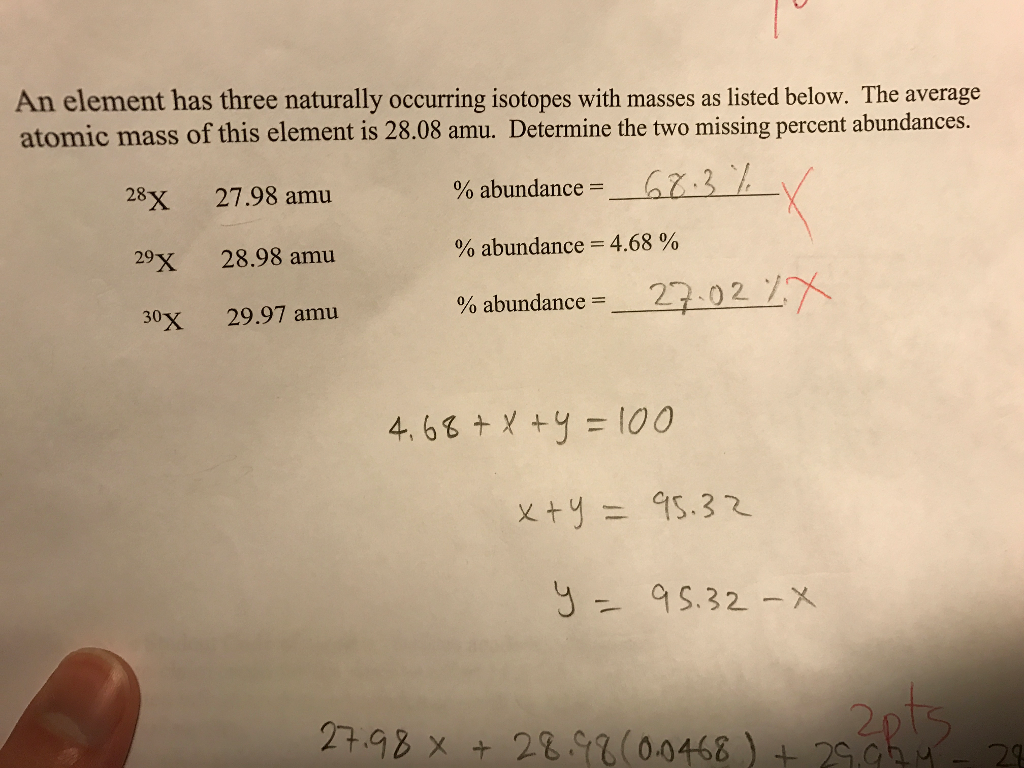An element has three naturally occurring isotopes with masses as listed below. The average atomic mass of this element is 28.08 amu. Determine the two missing percent abundances.^28X 27.98 amu % abundance =^29X 28.98 amu % abundance = 4.68 %^30X 29.97 amu % abundance =

• ### A certain element consists of two stable isotopes. The first has an atomic mass of 34.9689...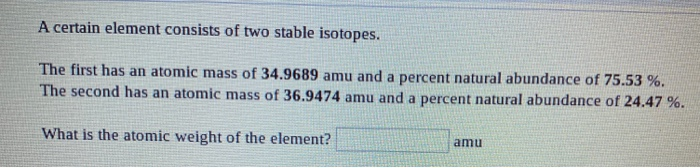A certain element consists of two stable isotopes. The first has an atomic mass of 34.9689 amu and a percent natural abundance of 75.53 %. The second has an atomic mass of 36.9474 amu and a percent natural abundance of 24.47 %. What is the atomic weight of the element? amu

• ### Given the following isotopes for oxygen and their percent abundance calculate oxygen's average atomic mass. (Show...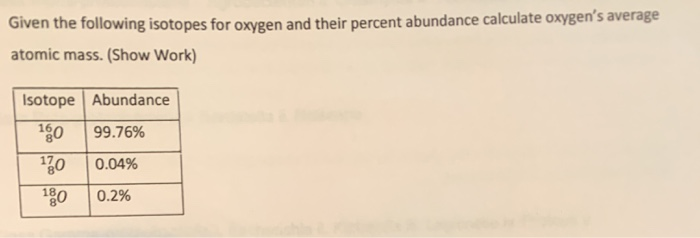Given the following isotopes for oxygen and their percent abundance calculate oxygen's average atomic mass. (Show Work) Isotope Abundance 160 99.76% 120 0.04% 0.2% 180

• ### The element lanthanum has an atomic weight of 139 and consists of two stable isotopes lanthanum-138...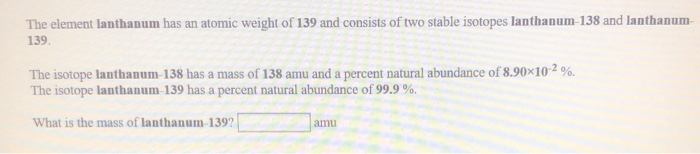The element lanthanum has an atomic weight of 139 and consists of two stable isotopes lanthanum-138 and lanthanum- 139. The isotope lanthanum-138 has a mass of 138 amu and a percent natural abundance of 8.90X10-2 %. The isotope lanthanum-139 has a percent natural abundance of 99.9%. What is the mass of lanthanum-1392 A certain element consists of two stable isotopes. The first has a mass of 113 amu and a percent natural abundance of 4.28 %. The second has a...

• ### In an imaginary universe, the element is composed of three isotopes. These isotopes, their exact masses,...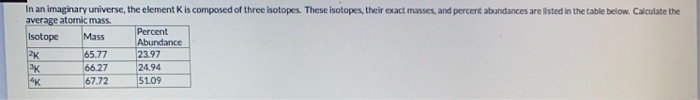In an imaginary universe, the element is composed of three isotopes. These isotopes, their exact masses, and percent abundances are listed in the table below. Calculate the average atomic mass. Percent Isotope Mass Abundance 2K 65.77 23.97 66.27 24.94 "K 67.72 51.09 SK

• ### The element oxygen consists of three naturally occuring isotopes: 16O, 17O, and 18O. The atomic mass...

The element oxygen consists of three naturally occuring isotopes: 16O, 17O, and 18O. The atomic mass of oxygen is 16.0 amu. What can be implied about the relative abundances of these isotopes?

• ### Chlorine has two isotopes. The lighter one has an atomic mass of 34.969 atomic mass units...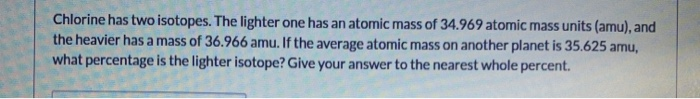Chlorine has two isotopes. The lighter one has an atomic mass of 34.969 atomic mass units (amu), and the heavier has a mass of 36.966 amu. If the average atomic mass on another planet is 35.625 amu, what percentage is the lighter isotope? Give your answer to the nearest whole percent.

• ### The element Oxygen consists of three naturally occurring isotopes with masses 15.949915, 16.999131, and 17.999159 amu....

The element Oxygen consists of three naturally occurring isotopes with masses 15.949915, 16.999131, and 17.999159 amu. The relative abundances of these three isotopes are 99.76, 0.03800, and 0.2000 percent, respectively. From these data calculate the average atomic mass of Oxygen (in amu).

• ### < Question 4 of 17 > Suppose that a fictitious element, X, has two isotopes: 59X...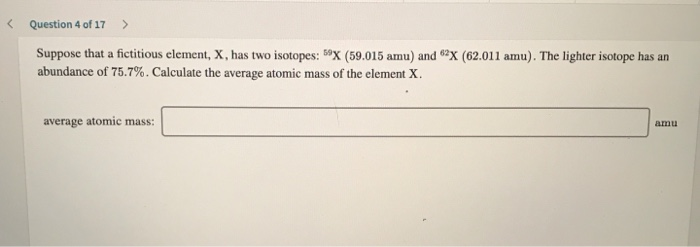< Question 4 of 17 > Suppose that a fictitious element, X, has two isotopes: 59X (59.015 amu) and "x (62.011 amu). The lighter isotope has an abundance of 75.7%. Calculate the average atomic mass of the element X average atomic mass:

• ### QUESTION 3 A fictitious element is composed of isotopes A and B with masses of 61.9887...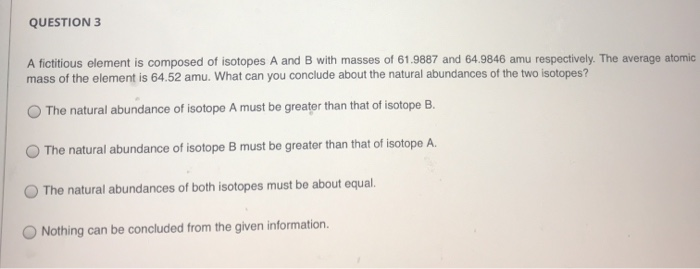QUESTION 3 A fictitious element is composed of isotopes A and B with masses of 61.9887 and 64.9846 amu respectively. The average atomic mass of the element is 64.52 amu. What can you conclude about the natural abundances of the two isotopes? O The natural abundance of isotope A must be greater than that of isotope B OThe natural abundance of isotope B must be greater than that of isotope A. O The natural abundances of both isotopes must be...